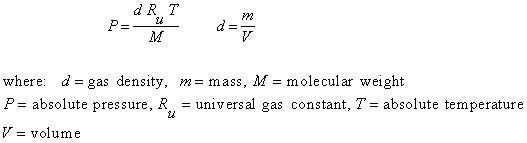Gas Specific Gravity and Ideal Gas Law Calculator

Gas Specific Gravity. Universal Gas Constant. Pressure, Temperature, Density, Mass, Volume using Ideal Gas Law.

Gas Specific Gravity and Molecular Weight Conversion (free)

 Select calculation: Compute M from S Compute S from M Gas Specific Gravity, S: Molecular Weight, M (g/mole): © 2014 LMNO Engineering, Research, and Software, Ltd. http://www.LMNOeng.com

Ideal Gas Calculator
Register to enable "Calculate" button.

 Solve for: Pressure (d, T, M known) Pressure (m, V, T, M known) Temperature (d, P, M known) Temperature (m, V, P, M known) Density (P, T, M known) Mass (P, T, V, M known) Volume (P, T, m, M known) Molecular Weight (P, T, d known) Molecular Weight (P, T, m, V known) Select gas: Computed or User-entered M Standard Air Ammonia, NH3 Argon, Ar Carbon Dioxide, CO2 Carbon Monoxide, CO Chlorine, Cl2 Fluorine, F2 Helium, He Hydrogen, H2 Hydrogen Sulfide, H2S Krypton, Kr Methane, CH4 Neon, Ne Nitrogen, N2 Nitric Oxide, NO Nitrous Oxide, N2O Oxygen, O2 Sulfur Dioxide, SO2 Xenon, Xe Molecular Weight, M (g/mole): Absolute Pressure, P: N/m2 or Pa bar atm torr or mm Mercury (0C) psi lb(force)/ft2 inch H2O (60F) inch Mercury (60F) Temperature, T: Celsius Kelvin Fahrenheit Rankine Density, d: kg/m3 g/cm3 slug/ft3 lb(mass)/ft3 Mass, m: kg Volume, V: m3 © 2014 LMNO Engineering, Research, and Software, Ltd. http://www.LMNOeng.com

Units in gas specific gravity and ideal gas calculators: atm=atmosphere, C=Celsius, cm=centimeter, F=Fahrenheit, ft=foot, g=gram, kg=kilogram, m=meter, mm=millimeter, N=Newton, Pa=Pascal, psi=pound per square inch.

Equations for Gas Specific Gravity and Molecular Weight Conversion

S = M / Mair , where S=gas specific gravity, M=gas molecular weight, Mair=28.96443 g/mole (molecular weight of standard air - CRC, 1983).

The gas specific gravity calculation does not check for unreasonable inputs. Please enter positive values.

Equations for Ideal Gas Law Calculator (CRC, 1983)Ru = 8.3144126  N-m/mole-K

The ideal gas law calculation internally converts all user inputs to SI units, performs the calculation, then converts calculated values to user-desired units. All molecular weights are from CRC (1983). The molecular weight of air is computed from the volume fractions of the individual components in air given by CRC (1983):

Fractional Volumes of Components in Sea Level Dry Air (CRC, 1983)

 Component: N2 O2 Ar CO2 Ne He Kr Xe CH4 H2 Volume Fraction 0.78084 0.209476 9.34e-3 3.14e-4 1.818e-5 5.24e-6 1.14e-6 8.7e-8 2e-6 5e-7

Error Messages given by Ideal Gas calculation
"Inputs must be positive".  This is an initial check of user input. The only negative values that may be input are temperature expressed in oC or oF.
"T must be > 0".  This message will appear if temperature is entered in oR or K.
"T must be > -273.15".  This message will appear if temperature is entered in oC. -273.15 oC corresponds to absolute zero.
"T must be > -459.67".  This message will appear if temperature is entered in oF. -459.67 oF corresponds to absolute zero.

References
Chemical Rubber Company (CRC).  1983.  CRC Handbook of Chemistry and Physics. Weast, Robert C., editor.  63rd edition.  CRC Press, Inc.  Boca Raton, Florida.  USA.

LMNO Engineering, Research, and Software, Ltd.
7860 Angel Ridge Rd.   Athens, Ohio 45701  USA   Phone: (740) 707-2614
LMNO@LMNOeng.com    http://www.LMNOeng.com

To: